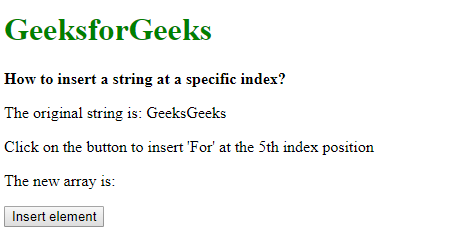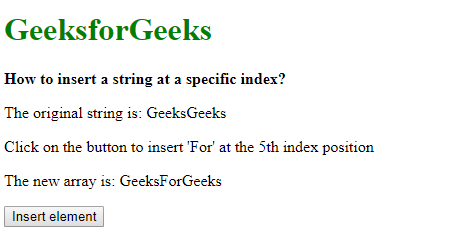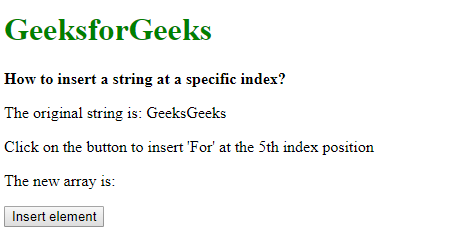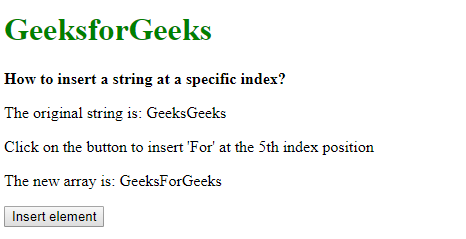Related Articles

# How to insert a string at a specific index in JavaScript ?

• Last Updated : 25 Sep, 2019

Given a string containing words and the task is to insert a new string at a given index. There are two methods to solve this problem which are discussed below:

Method 1: Using the slice() method: The string is first divided into two parts by splitting it where the new string has to be inserted. The slice() method is used to return an array between two indices. It takes two parameters, one is the beginning index which specifies and the other is the ending index.
The first part of the string is extracted using the starting position as 0 and the ending position as the index where the new string has to be inserted. The second part of the string is extracted using the starting position as index where the new string has to be inserted. The ending position is optional here and it will be assumed till the end of the string. The new string is added in between these two parts by simple string concatenation.

Example:

 ```<``html``>`` ` `<``head``>``    ``<``title``>``        ``How to insert a string``        ``at a specific index?``    `````` ` `<``body``>``    ``<``h1` `style``=``"color: green"``>``        ``GeeksforGeeks``    ````     ` `    ``<``b``>``        ``How to insert a string``        ``at a specific index?``    ````     ` `    ``<``p``>The original string is: GeeksGeeks``     ` `    ``<``p``>``        ``Click on the button to insert``        ``'For' at the 5th index position``    ````     ` `    ``<``p``>``        ``The new array is: ``        ``<``span` `class``=``"outputString"``>``    ```` ` `    ``<``button` `onclick``=``"insertString()"``>``        ``Insert element``    ````     ` `    ``<``script` `type``=``"text/javascript"``>``         ` `        ``function insertString() {``            ``let origString = "GeeksGeeks";``            ``let stringToAdd = "For";``            ``let indexPosition = 5;`` ` `            ``newString = origString.slice(0, indexPosition)``                    ``+ stringToAdd``                    ``+ origString.slice(indexPosition);`` ` `            ``document.querySelector('.outputString').textContent``                    ``= newString;``        ``}``    `````` ` ``

Output:

• Before clicking on the button:• After clicking on the button:Method 2: Using the splice() method: The split() method is used to split a string on the basis of a separator. This method can be used to split the string into individual letters by specifying a blank separator. This step is used to convert a string to an array as the next step requires it.
The splice() method is used to insert or replace contents of an array at a specific index. This can be used to insert the new string at the position of the array. It takes 3 parameters, the index where the string is to be inserted, the number of deletions to be performed if any, and the string to be inserted.
The required index and the string is passed as parameters to this method with the deletions parameter set to 0. It inserts the string into the array. The array is joined back using the join() method. This makes it one whole string again with the new string joined in between.

Example:

 ```<``html``>`` ` `<``head``>``    ``<``title``>``        ``How to insert a string``        ``at a specific index?``    `````` ` `<``body``>``    ``<``h1` `style``=``"color: green"``>``        ``GeeksforGeeks``    ````     ` `    ``<``b``>``        ``How to insert a string``        ``at a specific index?``    ````     ` `    ``<``p``>``        ``The original string``        ``is: GeeksGeeks``    ````     ` `    ``<``p``>``        ``Click on the button to insert``        ``'For' at the 5th index position``    ````     ` `    ``<``p``>``        ``The new array is: ``        ``<``span` `class``=``"outputString"``>``    ```` ` `    ``<``button` `onclick``=``"insertString()"``>``        ``Insert element``    ````     ` `    ``<``script` `type``=``"text/javascript"``>`` ` `        ``function insertString() {``            ``let origString = "GeeksGeeks";``            ``let stringToAdd = "For";``            ``let indexPosition = 5;`` ` `            ``// Split the string into individual``            ``// characters``            ``origString = origString.split('');`` ` `            ``// Insert the string at the index position``            ``origString.splice(indexPosition, 0, stringToAdd);`` ` `            ``// Join back the individual characters``            ``// to form a new string``            ``newString = origString.join('');`` ` `            ``document.querySelector('.outputString').textContent``                        ``= newString;``        ``}``    `````` ` ``

Output:

• Before clicking on the button:• After clicking on the button:My Personal Notes arrow_drop_up